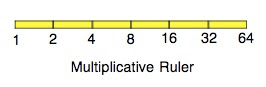Friday, February 22, 2008

Fun with Theory of Measurement

Suppose we have three sticks, and we want to know whether the first two, when stuck together end to end, are longer or shorter than the third. We all know that we can measure the first two sticks, add the numbers we get, and compare the sum to the number we get when we measure the third stick. Would it be possible to measure length in such a way that when we combine the lengths of the first two sticks we would multiply instead of adding?

The answer is, yes, it is possible. This picture shows the unusual kind of ruler we would use:This shows how it works:Notice that the null stick has length 1 with this ruler. A little thought shows that this must be true: the null stick is the identity for putting sticks together, and so its measure must be the identity for the operation we use for putting lengths together.

Now that you see you can use multiplication rather than addition to combine lengths, do you think we could use subtraction?

The answer is that we can't. Putting sticks together is commutative, where comparison is concerned: it doesn't matter which stick we start with when putting them together. But subtraction is not commutative. So we can't use subtraction to represent putting sticks together.